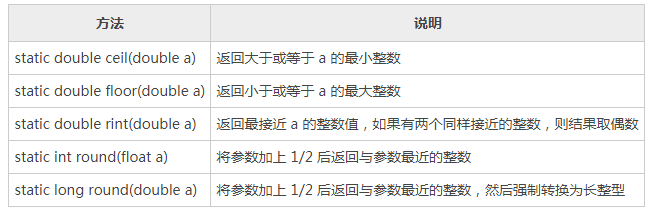# Java Math类的常用方法，求整运算

Math 类的求整方法有很多，详细说明如下所示：```import java.util.Scanner;
public class Test03
{
public static void main(String[] args)
{
Scanner input = new Scanner(System.in);
System.outprintln("请输入一个数字：");
double num = input.nextDouble();
System.out.println("大于或等于 " + num + " 的最小整数：" + Math.ceil(num));
System.out.println("小于或等于 " + num + " 的最大整数：" + Math.floor(num));
System.out.println("将 " + num + " 加上 0.5 之后最接近的整数：" + Math.round(num));
System.out.println("最接近 " + num + " 的整数：" + Math.rint(num));
}
}```

```请输入一个数字：
99.01

Java Math类的常用方法，求最大值、最小值和绝对值

Java Math类的常用方法，静态常量

java System类的成员变量，PrintStream out，InputStream in，PrintStream err详解